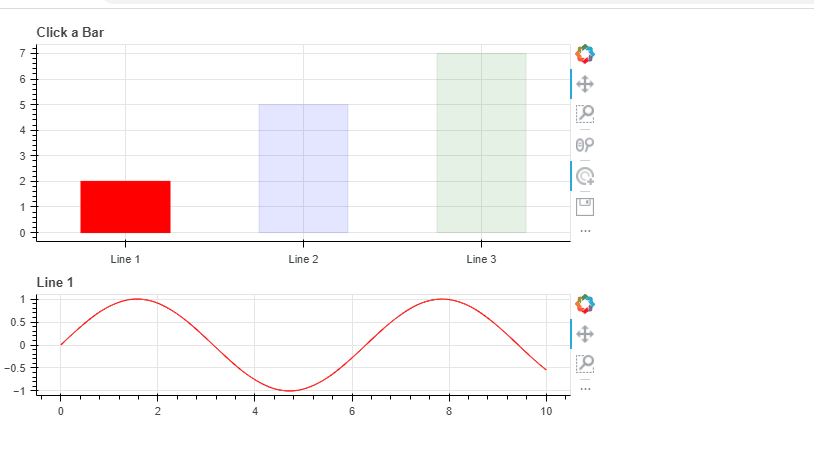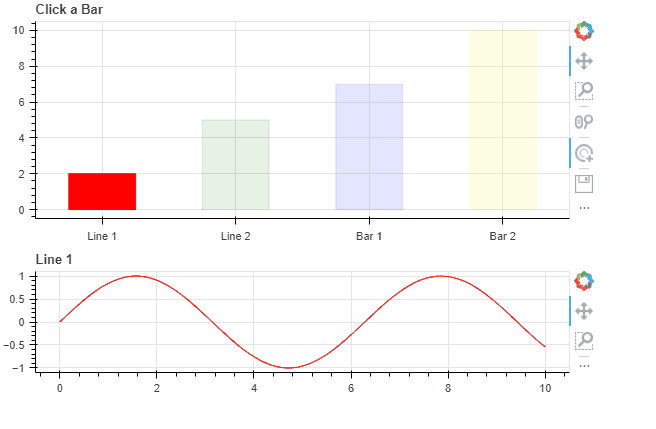# A different plot in another figure when a bar of a Barchart is clicked

Hi everybody,

I am trying to build with bokeh an interface for which the main plot is a barchart where every bar is associated with some other data that can be represented in a lineplot.

My idea is that whenever a user clicks on a specific bar, the specific lineplot figure (with the appropriate curve) appears just below the barchart.

If possible, I would even prefer that the line is changed when the mouse just hovers the bar, and the line eventually remains when the bar is clicked.

As a dummy example here’s some code below:

``````import bokeh
import numpy as np
from bokeh.io import output_notebook
from bokeh.plotting import figure, show
output_notebook()

from bokeh.layouts import gridplot

xx = np.linspace(0,10,500)
y = np.sin(xx)
barelements = [2,5,7]

figure_barchart = figure(height=250, title="A Barchart")
figure_barchart.vbar(x=[0,1,2], top=barelements, width=0.5, bottom=0, color="red")

p1 = figure(title='line1', height=150)
p1.line(x=xx, y=y)

p2 = figure(title='line2', height=150)
p2.line(x=xx*3, y=y**2)

p3 = figure(title='line3', height=150)
p3.line(x=xx/2, y=y**3)

show(gridplot([[figure_barchart],[p1],[p2],[p3]]), tools="")
``````

Which should produce something like this:

The details of the data used are not important, what I care about is that in the end I just have a column of 2 figures, where the one at the bottom changes whenever I click on a different bar of the barchart.

While in this example things are still manageable because I only have 3 bars, my use case could have in principle much many more bars (on the order of 50 if not more).

Do you have any suggestions about how I could go implementing this?

Hey, there are a lot of ways to do this, but here’s probably the simplest:

``````import numpy as np

from bokeh.plotting import figure, save
from bokeh.models import CustomJS, ColumnDataSource, TapTool

from bokeh.layouts import layout

xx = np.linspace(0,10,500)
y = np.sin(xx)
barelements = [2,5,7]

#create a dictionary for each bar element based the dummy data/equations you provided

#idea is that this dictionary will get passed to CustomJS and then used to replace the data in the datsource that's plotting the lines

src_dict = {'Line 1':{'x':xx,'y':y}
,'Line 2':{'x':xx*3,'y':y**2}
,'Line 3':{'x':xx/2,'y':y**3}}

#make a columndatasource for your bar
bar_src = ColumnDataSource({'x':list(src_dict.keys()),'y':barelements,'c':['red','blue','green']})

src=ColumnDataSource(data=src_dict['Line 1'])

figure_barchart = figure(height=250, title="Click a Bar",x_range=list(src_dict.keys()))
bar_rend = figure_barchart.vbar(x='x', top='y', width=0.5, bottom=0, color='c',source=bar_src)

p1 = figure(title='Line 1', height=150)

line_rend = p1.line(x='x', y='y',line_color='red',source=src)

#create the TapTool, add it to the bar figure, and enable it on initialization
tap = TapTool(renderers=[bar_rend])
figure_barchart.toolbar.active_tap = tap

cb = CustomJS(args=dict(bar_src=bar_src,src_dict=src_dict,src=src,p1=p1,line_rend=line_rend)
,code='''
//get the index of the selected bar
//getting 0th index cuz we just want on index value
var sel_bar_i = bar_src.selected.indices
// what is the line name associated with that index?
var line_id = bar_src.data['x'][sel_bar_i]
//now that we know that line id, we can update the line's datasource via the src_dict
src.data = src_dict[line_id]
//update the line renderer's color to match just for demo purposes
line_rend.glyph.line_color = bar_src.data['c'][sel_bar_i]
//update the line plot title too just for demo purposes
p1.title.text=line_id
src.change.emit()
p1.change.emit()
''')
#apply this callback to trigger whenever the indices of the selected glyph change
bar_src.selected.js_on_change('indices',cb)

save(layout([figure_barchart,p1]),'check.html')
``````My recommendation, big time, is to really study the CustomJS callback, write a bunch of console.log(NAMEOFSOMEVARIABLE/OBJECT) in it, and then look at the javascript console in the finished html to help you follow along.

The alternative way I might go about doing this would be to use a multiline glyph built from a single source of all lines and a customjs filter to filter out for only the one corresponding to the selected bar. I figured showing this with the “change the plotted datasource” approach a bit more straightforward.EDIT: originally uploaded the wrong gif…

2 Likes

Thank you very much @gmerritt123 , this works like a charm!!!

I now realised though that some bars are associated with a line (as here), but some others are associated with another barplot… Do you think it is possible to modify the code to incorporate this need?

That will probably get trickier for two reasons: 1) the src_dict will no longer be able to be “uniform” because your bar plots and line plots will need different arguments to drive them so the approach will have to be more generalized… and 2) The axis tick labels will need to toggle between categorical (i assume your bars hold categorical data?) and numerical (the line plots). I might find time to take a crack at it but don’t hold your breath1 Like

Alright, here’s a somewhat non-elegant solution. I broke the whole thing up into two different figures (one for bars with categorical x range, one for lines with numerical x range), and their visibility toggles based on the naming convention in src_dict. There is probably a way to get this all updating on one figure by instantiating FactorRange and CategoricalScale models and passing them to the callback, but this is more straightforward I think.

``````import numpy as np

from bokeh.plotting import figure, save
from bokeh.models import CustomJS, ColumnDataSource, TapTool
from bokeh.layouts import layout

xx = np.linspace(0,10,500)
y = np.sin(xx)
barelements = [2,5,7,10]

#create a source dictionary for each bar element based the dummy data/equations you provided
#idea is that this dictionary will get passed to CustomJS and then used to replace the data in the datsource that's plotting the lines and/or bars
src_dict = {'Line 1':{'x':xx,'y':y,}
,'Line 2':{'x':xx*3,'y':y**2}
,'Bar 1':{'x':['Apple','Orange','Banana'],'y':[5,6,7]} #new entry --> categorical data for a bar plot
,'Bar 2':{'x':['Onion','Carrot','Potato','Tomato'],'y':[2,10,15,10]} #new entry --> categorical data for a bar plot
}
colors = ['red','green','blue','yellow']
color_dict = {k:colors[i] for i,k in enumerate(src_dict.keys())}

#make a columndatasource for the "main" bar plot
bar_src = ColumnDataSource({'x':list(src_dict.keys()),'y':barelements,'c':colors})

figure_barchart = figure(height=250, title="Click a Bar",x_range=list(src_dict.keys()))
main_bar_rend = figure_barchart.vbar(x='x', top='y', width=0.5, bottom=0, color='c',source=bar_src)

#now it gets different. I set this up to have two different figures, one to show lines, the other bar.
#only one of these will be visible
line_fig = figure(title='Line 1', height=150)
bar_fig = figure(title='Bar 1',height=150
,x_range=src_dict['Bar 1']['x'] #initializing the x_range with Bar 1's categorical 'x'
)
#since we're starting with Line 1 showing, make bar_fig not visible initially
bar_fig.visible=False

#still can use on datasource, initialized with Line 1
src = ColumnDataSource(src_dict['Line 1'])
#renderer for the line on the line fig
line_rend = line_fig.line(x='x', y='y',line_color=color_dict['Line 1'],source=src)
#renderer for the bar on the bar fig
bar_rend = bar_fig.vbar(x='x',top='y',width=0.5, bottom=0, fill_color=color_dict['Bar 1'],source=src)

#create the TapTool, add it to the main bar figure, and enable it on initialization
tap = TapTool(renderers=[main_bar_rend])
figure_barchart.toolbar.active_tap = tap

#note the revised args here
cb = CustomJS(args=dict(bar_src=bar_src
,line_fig=line_fig, line_rend = line_rend
,bar_fig=bar_fig, bar_rend = bar_rend
,src=src, src_dict=src_dict
,color_dict = color_dict)
,code='''
//get the index of the selected bar
//getting 0th index cuz we just want on index value
var sel_bar_i = bar_src.selected.indices
// what is the name associated with that index?
var name = bar_src.data['x'][sel_bar_i]
//now that we know that name...
//based on your src_dict naming convention, you can flag which figure you want visible
if (name.split(' ') == 'Line'){
line_fig.visible = true
line_fig.title.text = name
bar_fig.visible = false
line_rend.glyph.line_color = color_dict[name]
}
else if (name.split(' ') == 'Bar'){
line_fig.visible = false
bar_fig.visible = true
bar_fig.title.text = name
bar_rend.glyph.fill_color = color_dict[name]
//extra piece here: update the factors on the x range to autoscale the x axis to fit the changed categorical data
bar_fig.x_range.factors = src_dict[name]['x']
}
src.data = src_dict[name]
src.change.emit()
line_fig.change.emit()
bar_fig.change.emit()
''')
#apply this callback to trigger whenever the indices of the selected glyph change
bar_src.selected.js_on_change('indices',cb)
lo = layout([figure_barchart,line_fig,bar_fig])

save(lo,'check.html')
``````1 Like

Hackerman!!!

Thank you so much!!!

Thank you for your time and for the quick response, I’m very grateful.

I’m more and more realising that I have to learn Javascript…

If you go the bokeh-server route you can avoid a lot of the JS and use python functions as callbacks instead. However for my work, being able to pass around standalone interactive htmls in lieu of static report figures etc. is the main attraction, so I’ve primarily learned the CustomJS route for things.

… I often post “hard/impossible to me” questions here and have been super lucky to have the brilliant core devs (the real “hackers” here) help me out. I’m just trying to give back by providing support for others in kind when I actually can solve their problem1 Like

This topic was automatically closed 90 days after the last reply. New replies are no longer allowed.